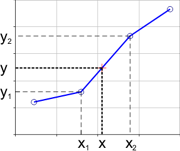## FANDOM

225 PagesLinear interpolation is a straight line fit between two data points.

## About

Linear Interpolation is a method for estimating the value of a function between two known values or within two plotted points. Linear interpolation involves estimating a new value by connecting two adjacent known values with a straight line.

If the two known values are (x1, y1) and (x2, y2), then the y value for some point x is:

$y = y1 + (x - x1) [(y2-y1) / (x2 - x1)]$